Benjamin Eysenbach,   Tianjun Zhang,   Ruslan Salakhutdinov,   Sergey Levine

Paper,   Code,   Video

tldr: We reframe goal-conditioned RL as the problem of predicting and controlling the future state distribution of an autonomous agent. We solve this problem indirectly by training a classifier to predict whether an observation comes from the future. Importantly, an off-policy variant of our algorithm allows us to predict the future state distribution of a new policy, without collecting new experience. While conceptually similar to Q-learning, our approach provides a theoretical justification for goal-relabeling methods employed in prior work and suggests how the goal-sampling ratio can be optimally chosen. Empirically our method outperforms these prior methods.

# Videos of Learned Policies

Below, we visualize examples of the behavoir learned by our method. We emphasize that our method does not use any reward shaping or hand-crafted distance functions. Note that the robot has learned to retry if it initially fails to solve the task, and has learned to finely adjust the position of the objects if its initial attempt slightly missed the goal.

### Sawyer Pushing

In this task, the robot is supposed to move the red puck to the goal, which is indicated by the green circle.

### Sawyer Pick

In this task, the robot is supposed to pick up the puck and lift it to the green circle.

### Sawyer Window

In this task, the robot is supposed to slide the window so that the handle is aligned with the green circle.

### Sawyer Drawer

In this task, the robot is supposed to pull or push the drawer so the handle is aligned with the green circle.

### Sawyer Faucet

In this task, the robot is supposed to turn the faucet to the handle is aligned with the (tiny) green circle.

### Sawyer Push Two

In this task, the robot is supposed to push two pucks to their respective goals (green goal for green puck, red goal for red puck).

# Predictions of the Future State Distribution

Our method predicts where an agent is likely to be in the time-discounted future. Below, we give our model an observation (top) and action (not shown) and it predicts a distribution over future states (bottom). As expected, the model predicts more distant states when we increase the discount factor $$\gamma$$.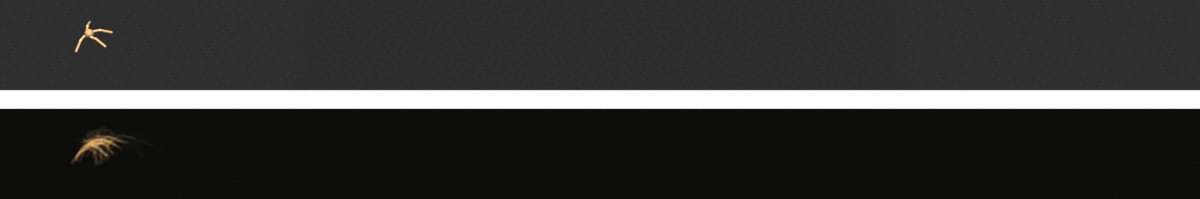Ant-v2, $$\gamma = 0.5$$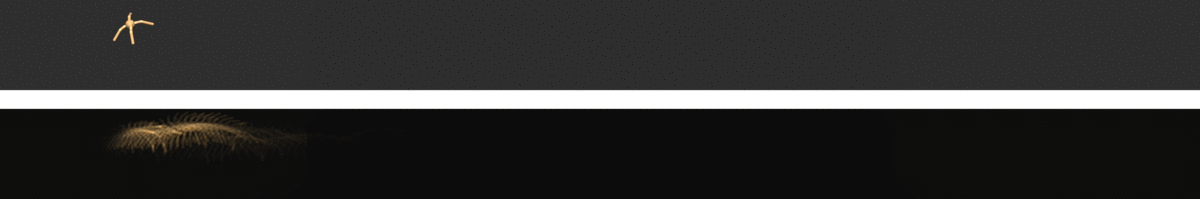Ant-v2, $$\gamma = 0.9$$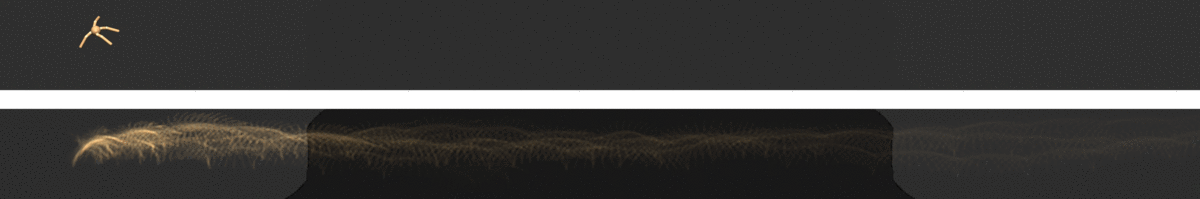Ant-v2, $$\gamma = 0.99$$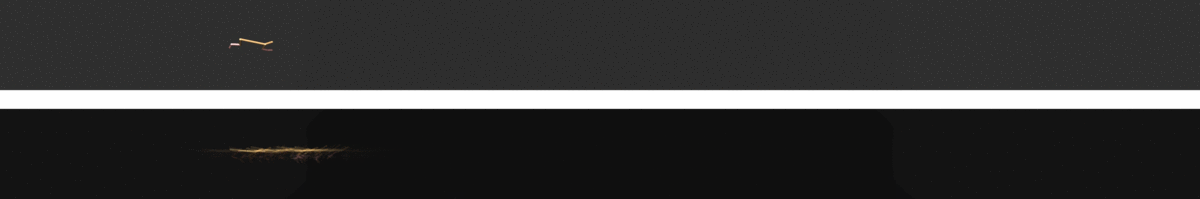HalfCheetah-v2, $$\gamma = 0.50$$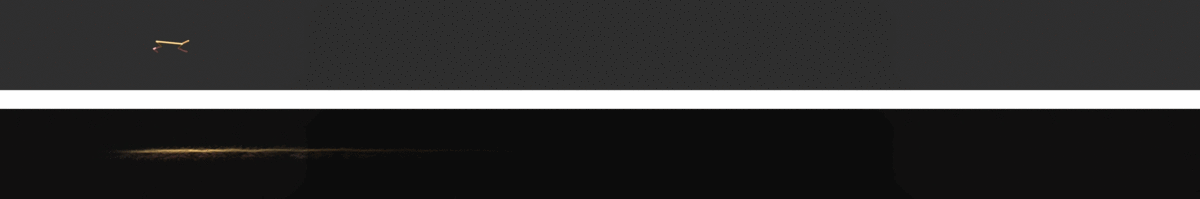HalfCheetah-v2, $$\gamma = 0.90$$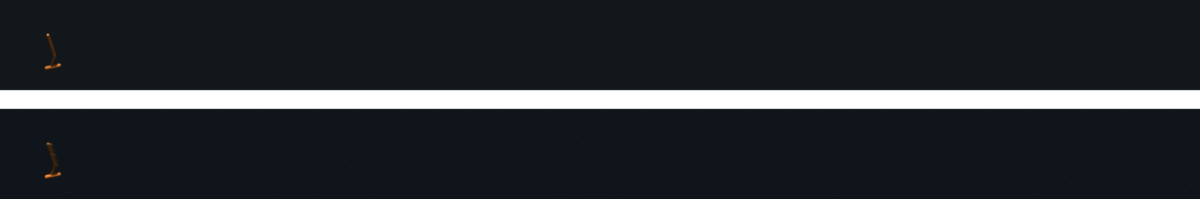Hopper-v2, $$\gamma = 0.50$$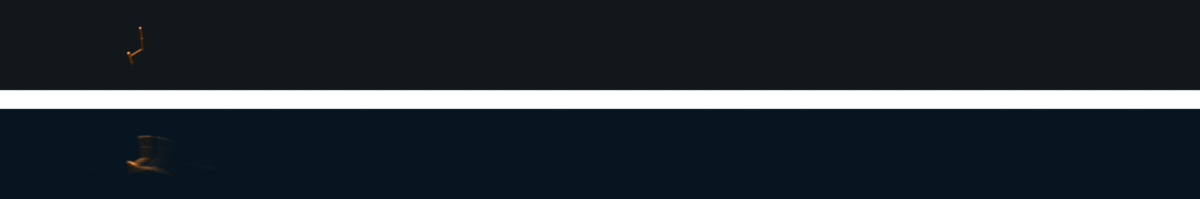Hopper-v2, $$\gamma = 0.90$$Walker-v2, $$\gamma = 0.50$$Walker-v2, $$\gamma = 0.90$$Walker-v2, $$\gamma = 0.99$$